How to know what kit is right for your child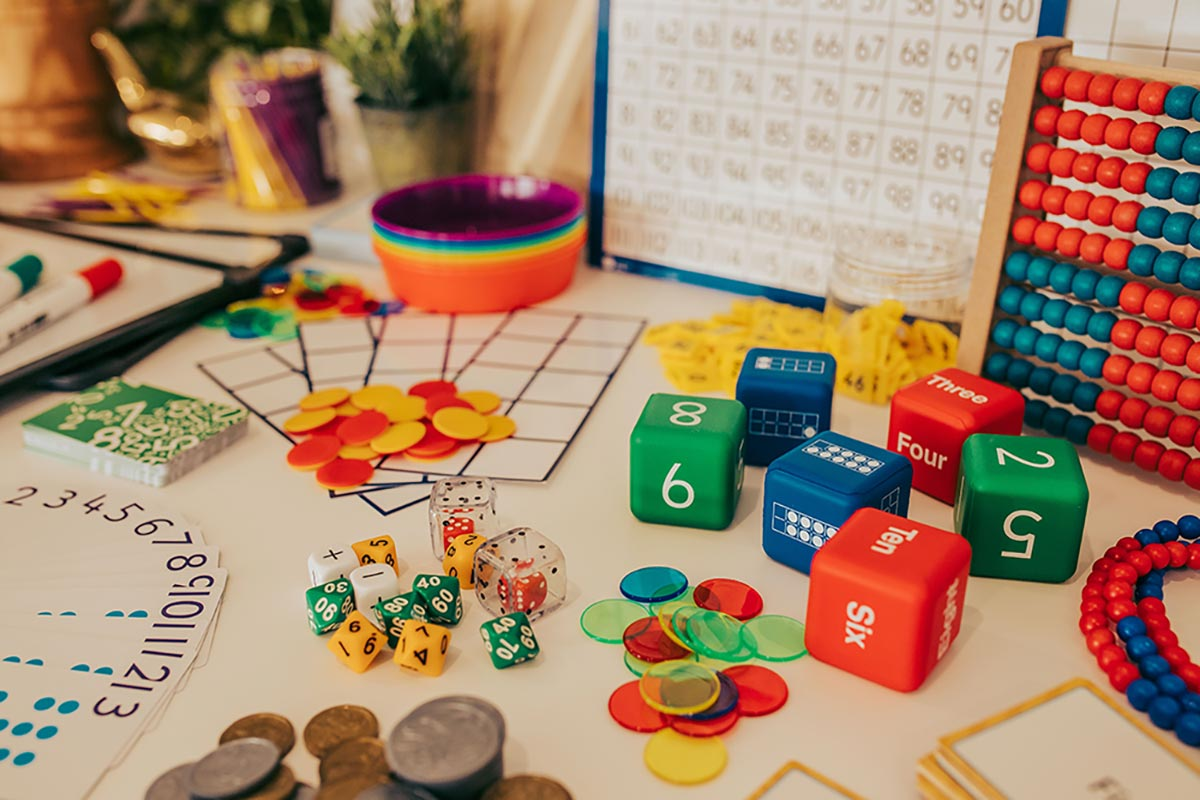3 Steps
Let’s Get Started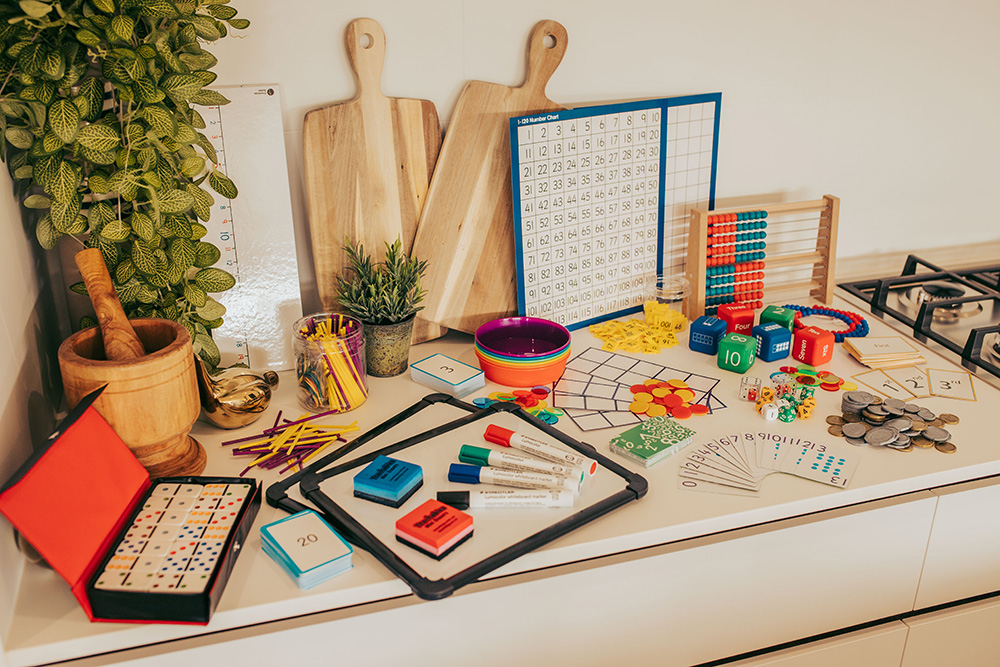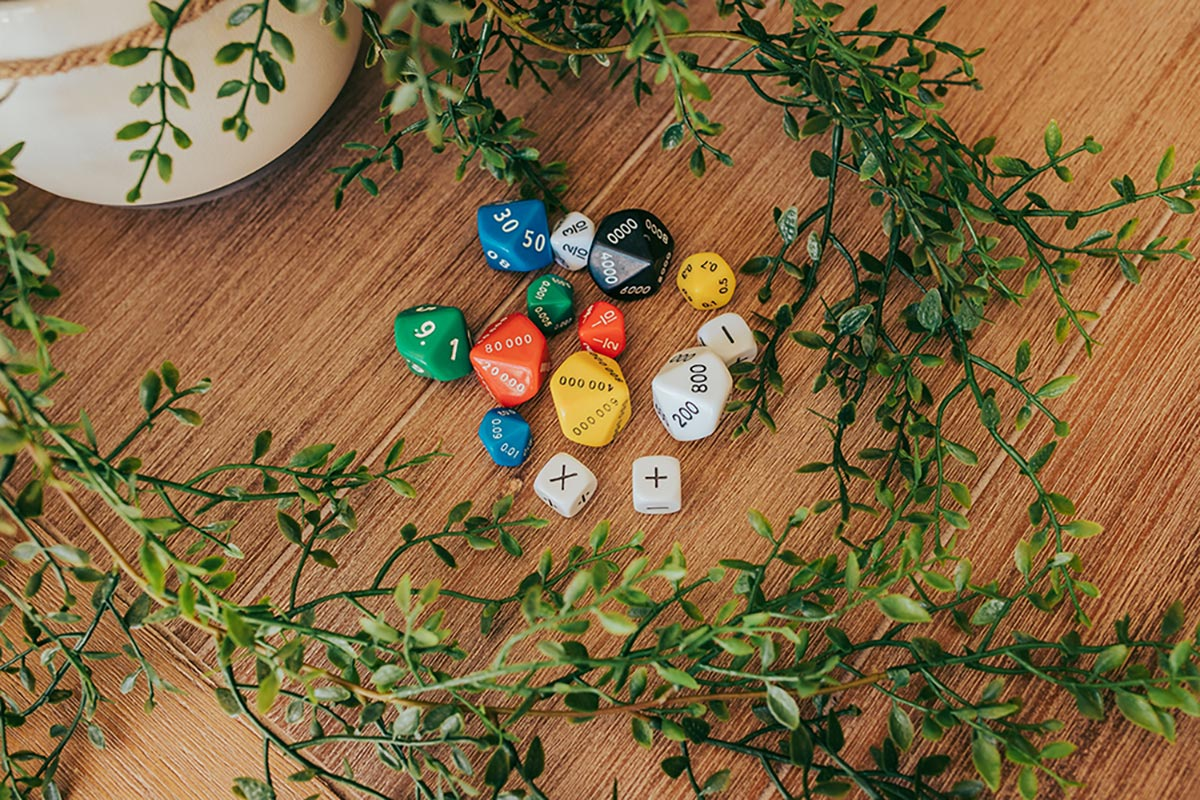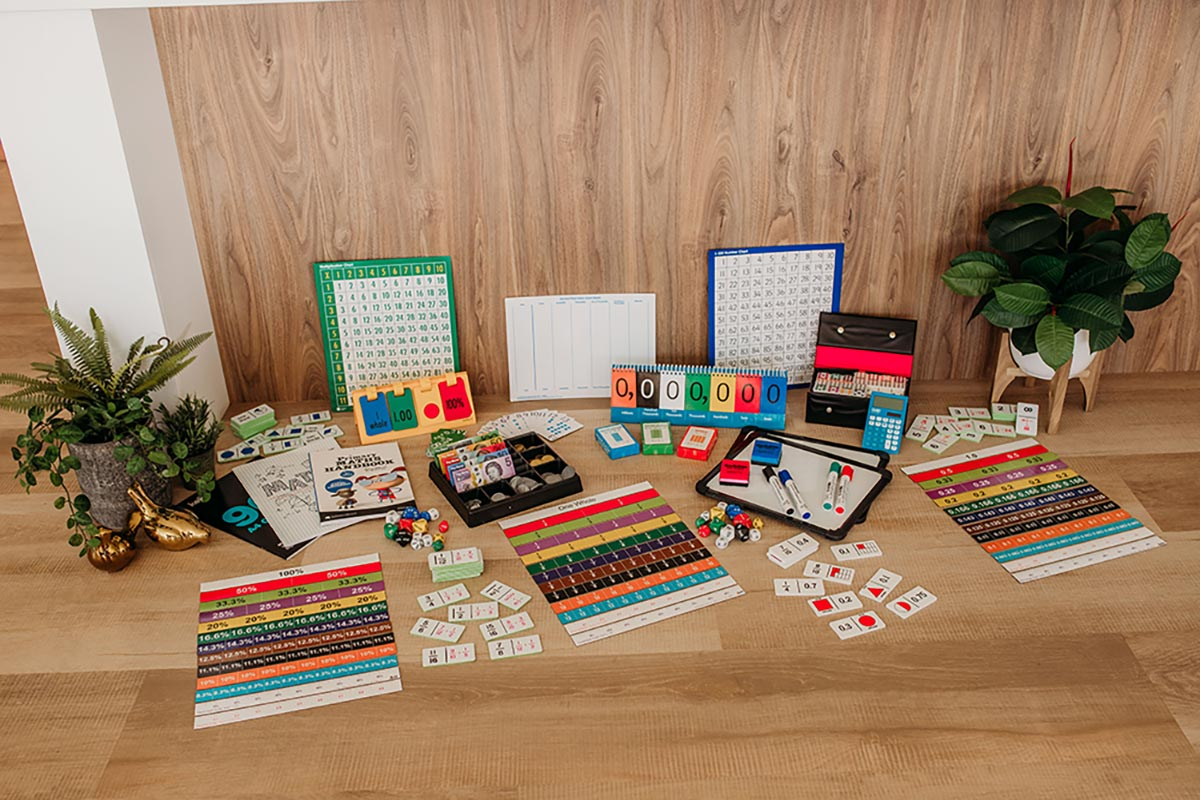Explore and be in awe!
How to choose the right kit?

Please refer to the table below outlining each kit and their learning outcomes

•#### How old is your child and what grade are they in at school?

If your child is not enrolled in school yet, our 0 – 10 Number Kit is the correct kit for you.

•#### How is your child achieving in their current grade at school?

• You can refer to their most recent school report
Most schools have an A-E academic achievement section for Mathematics.
A or B – Usually means they are working above stage expectations
C – Usually means they are achieving stage expectations
D or E – Usually means working below or significantly below stage expectations

The Mathematics comment section should provide greater insight into what your child can and can not do mathematically. Try to look for comments explaining how far they can count, what numbers they can read and write, what type of addition and subtraction sums they are completing in class and what strategies they are using.

• You can contact your child’s teacher and ask them specifically what they are learning in class and how your child is going.

•#### Ask your child specific questions to try and determine what they can and can not do.

•### Learning Outcomes and Expectations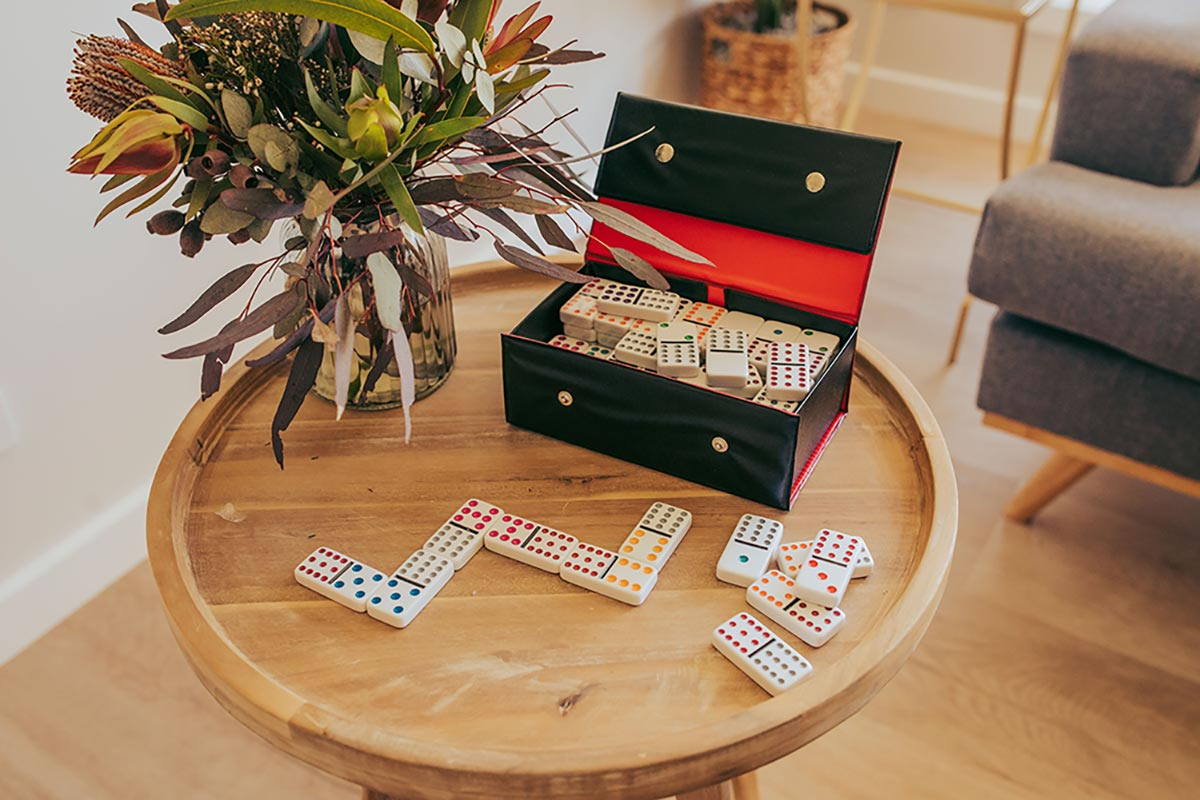0 - 10
Prior to School Learning
3 to 5 years of age

• Count forwards and backwards to 10

• Count and instantly recognise dot patterns to 10

• Read numerals 0 to 10

• Write numerals 0 to 10

• Identify numerals before and after a given number to 10

• Add two or more groups together

### Learning Outcomes and Expectations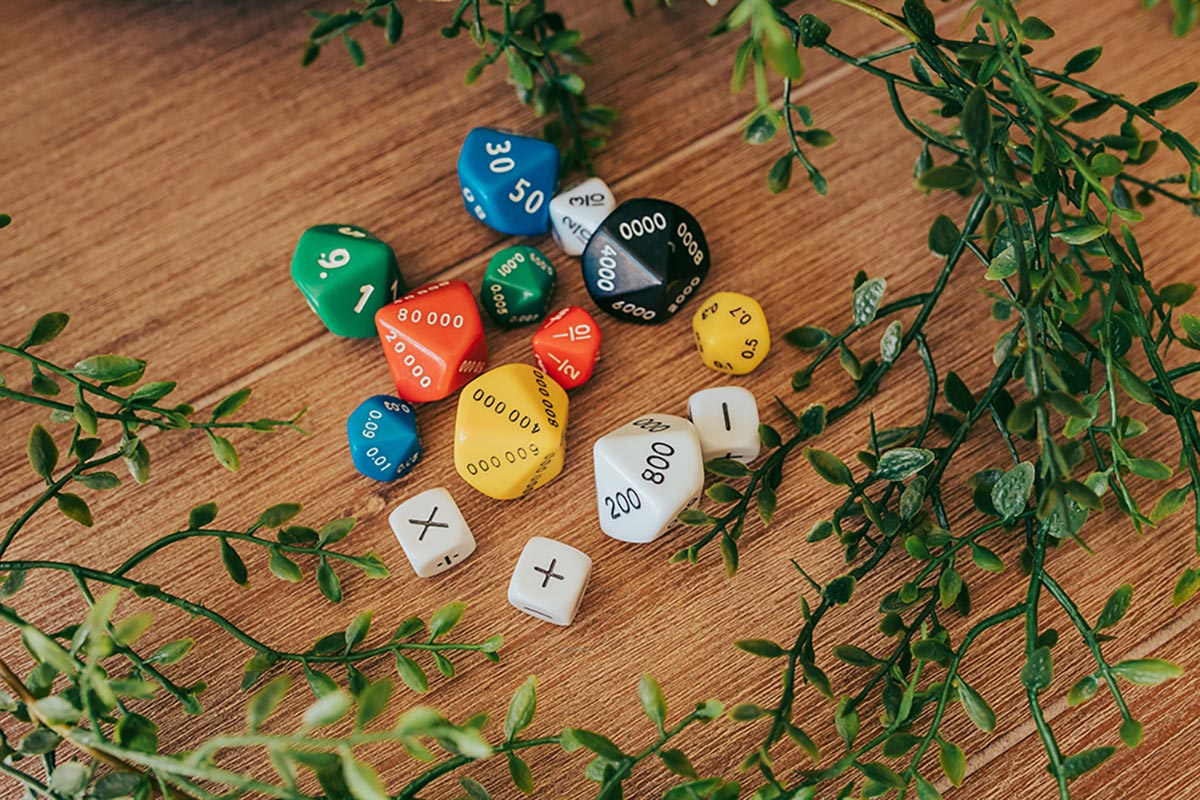0 - 20
Foundation/Kindergarten Expectation
5 to 6 years of age

• Count forwards to 30

• Count backwards from 20

• Count and instantly recognise dot patterns to 10

• Read numerals 0 to 20

• Write numerals 0 to 20

• Identify numerals before and after given numbers to 20

### Learning Outcomes and Expectations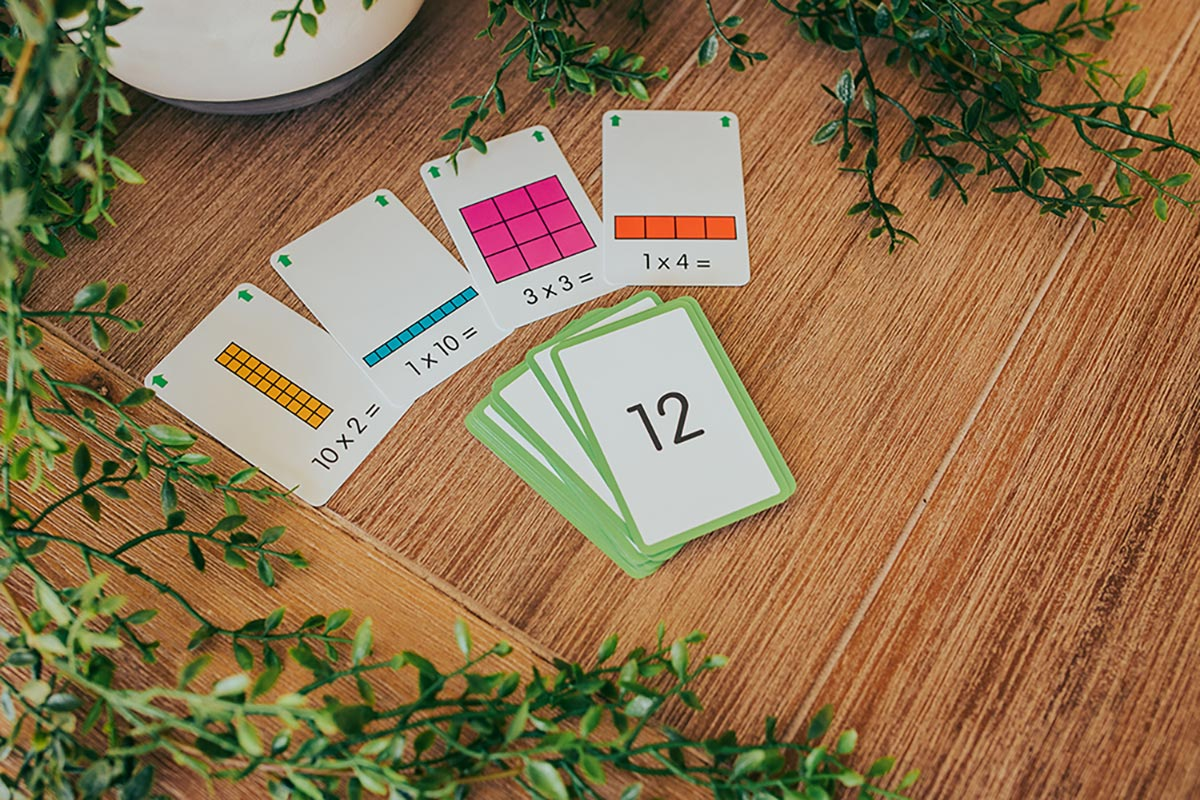0 - 120
Year One Expectation
6 to 7 years of age

• Count forwards and backwards by ones from any number from 0 to 120

• Skip count by twos, fives and tens starting from zero

• Read, write and order two-digit numbers

• Locate numbers on a number line to 120

• Count collections to 100 by partitioning two-digit numbers using place value

• Read and use ordinal numbers to 30th

• Recognise and describe one-half as one of two equal parts of a whole

• Represent and solve simple addition and subtraction problems using a range of strategies including counting on and partitioning. Eg 12 – 3 = 9, 11 + 5 =16

• Recognise, describe and order Australian coins according to their value

• Investigate and describe number patterns and patterns with objects

### Learning Outcomes and Expectations0 - 1000
Year Two Expectation
7 to 8 years of age

• Count forwards and backwards by twos, fives and tens from any starting point 0 to 120

• Read, write and order numbers to at least 1000

• Group, partition and rearrange collections up to 1000 in hundreds, tens and ones

• Solve simple addition and subtraction problems using a range of mental and written strategies for two digit numbers Eg 45 + 32 = 77, 68 – 34 = 34

• Recognise and represent multiplication as repeated addition, groups and arrays

• Recognise and represent division as grouping into equal sets

• Recognise and interpret common uses of halves, quarters and eighths of shapes and collections

• Count and order small collections of Australian coins and notes according to their value

• Describe patterns with numbers and identify missing elements

### Learning Outcomes and Expectations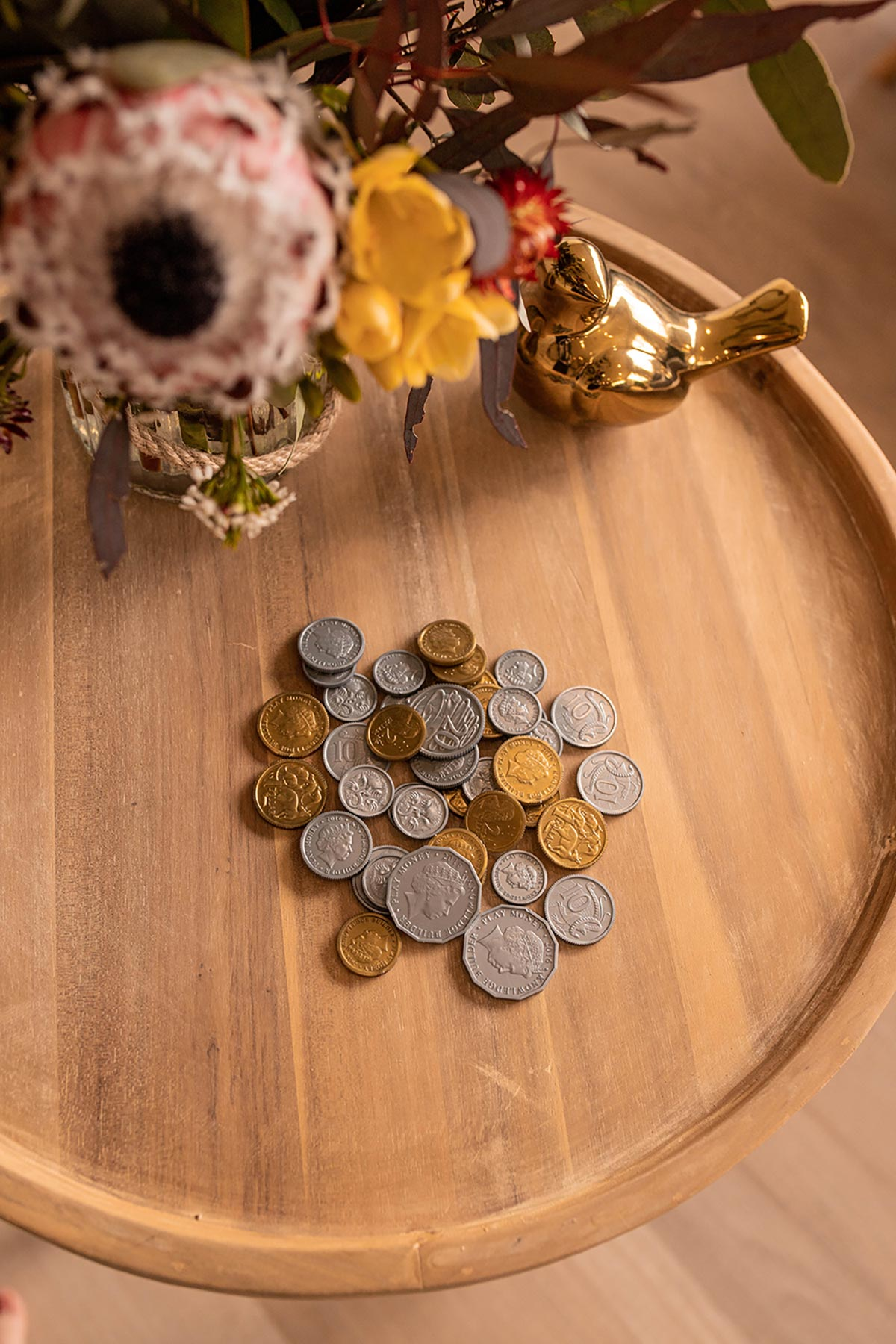0 - 1,000,000 +
Year Three to Year Six Expectation
8 to 12 years of age

• Investigate odd and even numbers

• Read, write and order numbers to at least 10,000, then tens of thousands and beyond

• Apply place value to at least 10,000, then tens of thousands

• Recall simple addition and subtraction facts to increase mental computation

• Solve 3,4,5-digit addition and subtraction sums using a range of mental and written strategies. Eg 135 + 426 = 561, 2463 – 1321 = 1142, 88765 +11223 = 99,988

• Investigate number sequences involving multiples of 3, 4, 6, 7, 8, and 9

• Recall multiplication facts to 10 x 10 and related division facts

• Solve multiplication and division problems

• Identify and describe factors and multiples of whole numbers

• Identify and describe properties of prime, composite, square and triangular numbers

• Investigate integers and locate and represent these numbers on a number line

• Model and represent unit fractions including ½, ¼, 1/3, 1/5 and their multiples to a complete whole

• Count by quarters, halves and thirds, including with mixed numerals

• Investigate equivalent fractions

• Compare and order common unit fractions and fractions with related denominators

• Solve addition and subtraction of fractions with the same or related denominator

• Recognise that the place value system can be extended to tenths, hundredths and beyond

• Compare, order and represent decimals

• Multiply decimals by whole numbers and perform divisions by non-zero whole numbers

• Multiply and divide decimals by powers of 10

• Represent money values in multiple ways

• Solve problems involving purchases and the calculation of change to the nearest five cents

• Calculate percentage discounts of 10%, 25% and 50% on sale items

• Describe number patterns as the result from addition, subtraction and multiplication

• Explore the use of brackets and order of operations to write number sentences

• Find unknown quantities in number sentences involving addition, subtraction, multiplication and division

• Identify equivalent number sentences involving addition, subtraction, multiplication and division

Keep up to date with the latest from the Educational Kits For Home team, by subscribing below.